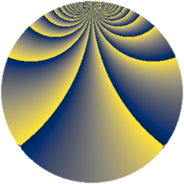# Properties

 Label 2001.2.bdLevel $2001$ Weight $2$ Character orbit 2001.bd Rep. character $\chi_{2001}(160,\cdot)$ Character field $\Q(\zeta_{28})$ Dimension $1440$ Sturm bound $480$

# Related objects

## Defining parameters

 Level: $$N$$ $$=$$ $$2001 = 3 \cdot 23 \cdot 29$$ Weight: $$k$$ $$=$$ $$2$$ Character orbit: $$[\chi]$$ $$=$$ 2001.bd (of order $$28$$ and degree $$12$$) Character conductor: $$\operatorname{cond}(\chi)$$ $$=$$ $$667$$ Character field: $$\Q(\zeta_{28})$$ Sturm bound: $$480$$

## Dimensions

The following table gives the dimensions of various subspaces of $$M_{2}(2001, [\chi])$$.

Total New Old
Modular forms 2928 1440 1488
Cusp forms 2832 1440 1392
Eisenstein series 96 0 96

## Trace form

 $$1440q - 8q^{2} + 8q^{8} + O(q^{10})$$ $$1440q - 8q^{2} + 8q^{8} + 224q^{16} + 8q^{18} + 4q^{23} - 320q^{25} + 24q^{26} + 56q^{29} + 48q^{31} - 256q^{32} + 112q^{35} + 240q^{36} + 16q^{39} - 8q^{41} + 48q^{46} - 88q^{47} + 224q^{48} + 336q^{49} - 16q^{50} - 224q^{52} + 56q^{55} - 24q^{58} - 16q^{59} + 56q^{69} - 152q^{70} + 112q^{71} + 64q^{72} - 40q^{73} + 16q^{75} - 56q^{77} + 240q^{81} + 48q^{82} - 128q^{85} + 8q^{87} - 32q^{94} - 16q^{95} - 144q^{98} + O(q^{100})$$

## Decomposition of $$S_{2}^{\mathrm{new}}(2001, [\chi])$$ into newform subspaces

The newforms in this space have not yet been added to the LMFDB.

## Decomposition of $$S_{2}^{\mathrm{old}}(2001, [\chi])$$ into lower level spaces

$$S_{2}^{\mathrm{old}}(2001, [\chi]) \cong$$ $$S_{2}^{\mathrm{new}}(667, [\chi])$$$$^{\oplus 2}$$